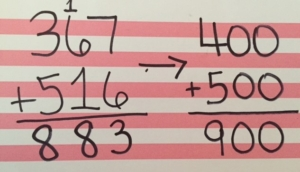What is estimation?
Estimating is an important skill. Estimation is the process of getting an approximate amount, sum or difference. We want students to be able to use mental math to more quickly to arrive at a reasonable answer for their sum or difference. In 3rd grade, students are adding and subtraction fluently within 1,000.

What are different ways to estimate?
• Front-end estimation is a reasonable strategy to estimate sums and differences when all or most of the numbers have the same number of digits. To use this strategy, add or subtract the front digits of the number (or the digits in the greatest place), then make adjustments based on the numbers in the next greatest place.

Example: 345 + 416 + 298. Add the 3, 4, and 2 hundreds (numbers in the greatest place) to get an estimate of 900. Adjust by adding the numbers in the next greatest place which are 4, 1, and 9 tens to get 140. 900 + 140 gives you a front-end estimation of 1,040.

• Compatible numbers is a useful strategy when adding more than 2 addends, or typically a long list of numbers. With this strategy, you look for 2 or 3 numbers that can be combined to create 10 or 100 to make it easier to determine an estimate.

Example: When adding 18 + 39 + 71 + 27 + 78 + 62, I can estimate the sum as 300 by combining numbers that estimate to 100: 18 and 78; 39 and 62; 71 and 27.

• Rounding is used to determine the nearest 10 or 100 to the whole number to estimate a sum or difference, many times to give you closer approximation.

Example: 328 + 74. Rounding to the nearest ten I estimate 330 + 70 = 400.

Why is it important to estimate sums and differences?
Estimation is an important skill to utilize without being prompted. It helps students determine reasonableness of their solution. This should occur before students actually solve for the sum or difference, and then compare the actual solution to the estimate students need to solve again for accuracy. Without estimation skills, students aren’t able to determine if their answer is within a reasonable range.

Estimation is also an important life skill. When you are shopping and you want to stay within a budget, you are more likely to estimate the cost of all the items you want to purchase than to pull out a calculator to determine the exact amount, including taxes while standing in the store. We want students to see important connections to math and everyday life. This happens in the classroom as we connect real-world using problem solving scenarios.

How do I help my students/child explore and develop an understanding of estimating sums and differences?
• Practice estimating whole numbers by using a number line.
The use of a number line is essential when exploring estimating sums and differences. You want to begin with students determining the range for one of the numbers involved. Then determine the middle number. From there, place the location of the number on the number line. Determine which of the range numbers the whole number is closest to, that becomes the estimated number.

For example: Below is an example of estimating 37. The student determined the range of 30-40 for the number line. The middle number is 35. The location for 37 was placed and it was closer to 40. That means 37 is estimated to be 40.This can also be done by pre-making different ranges of number lines on index cards. Place a number under the elmo or on the board and have students “put their heads together” to determine and justify which range would be used to estimate the given number, what the middle number would be, what each individual number is estimated, based on the numbers’ location on the number line, and the estimated sum or difference.

Below is an example of possible number lines to estimate the given addition problem.The range of 300-400 was selected for the number 367. The range of 500-600 was selected for the number 516. From there the student determine the middle numbers and the location of each of the numbers on that number line to determine 367 is estimated to 400, and 516 is estimated to 500.

From there, the students adds the two numbers to estimate the sum at 900. When the student actually finds the sum of the original addition problem, they can see that their estimate is reasonable.• Apply the estimation to determining sums and differences as a strategy for determining reasonableness of an answer.
After students understand the concept of estimating, have them apply that understanding to addition and subtraction problems. Students need to understand that it is a necessary step to determining if their end solution is reasonable, and should continue to use this skill even when it’s not prompted to do so.Eventually students will get to the point where this can happen mentally as shown in the example below.• Solve problems that require estimation as the answer.
Students need to understand that sometimes the estimated sum or difference becomes the answer. They don’t need to solve for the actual solution with these problems.

An example problem may include, “Tonya has \$812 in her checking account. She wants to go shopping to purchase a purse for \$316 and a pair of jeans for \$78. Does she have enough money? If so, about how much will she have left in her checking account?”

Read the article located at http://goo.gl/x3JviT for more information on why it’s important to focus on the skill of estimation with students.

Explore the virtual manipulatives Place Value Number Line. This manipulative requires students to drag a value to its appropriate place on a number line. The endpoints of the number line can be adjusted using the place tab in the bottom left corner of the screen. Students can use this tool to identify “nice” or compatible numbers.